# How to calculate time in Excel - time difference, adding / subtracting times

This tutorial focuses on various ways to calculate times in Excel. You will find a few useful formulas to add and subtract times, calculate time difference, or elapsed time, and more.

In the last week's article, we had a close look at the specificities of Excel time format and capabilities of basic time functions. Today, we are going to dive deeper into Excel time calculations and you will learn a few more formulas to efficiently manipulate times in your worksheets.

## How to calculate time difference in Excel (elapsed time)

To begin with, let's see how you can quickly calculate elapsed time in Excel, i.e. find the difference between a beginning time and an ending time. And as is often the case, there is more than one formula to perform time calculations. Which one to choose depends on your dataset and exactly what result you are trying to achieve. So, let's run through all methods, one at a time.

#### Formula 1. Subtract one time from the other

As you probably know, times in Excel are usual decimal numbers formatted to look like times. And because they are numbers, you can add and subtract times just as any other numerical values.

The simplest and most obvious Excel formula to calculate time difference is this:

=End time - Start time

Depending on you data structure, the actual time difference formula may take various shapes, for example:

 Formula Explanation `=A2-B2` Calculates the difference between the time values in cells A2 and B2. `=TIMEVALUE("8:30 PM") - TIMEVALUE("6:40 AM")` Calculates the difference between the specified times. `=TIME(HOUR(A2), MINUTE(A2), SECOND(A2)) - TIME(HOUR(B2), MINUTE(B2), SECOND(B2))` Calculates the time difference between values in cells A2 and B2 ignoring the date difference, when the cells contain both the date and time values.

Remembering that in the internal Excel system, times are represented by fractional parts of decimal numbers, you are likely to get the results similar to this: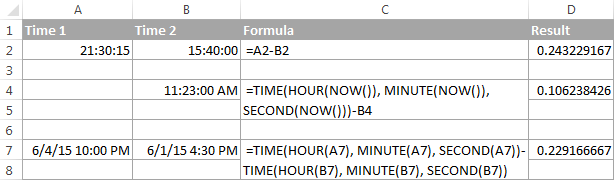The decimals in column D are perfectly true but not very meaningful. To make them more informative, you can apply custom time formatting with one of the following codes:

 Time code Explanation h Elapsed hours, display as 4. h:mm Elapsed hours and minutes, display as 4:10. h:mm:ss Elapsed hours, minutes and seconds, display as 4:10:20.

To apply the custom time format, click Ctrl + 1 to open the Format Cells dialog, select Custom from the Category list and type the time codes in the Type box. Please see Creating a custom time format in Excel for the detailed steps.

And now, let's see how our time difference formula and time codes work in real worksheets. With Start times residing in column A and End times in column B, you can copy the following formula in columns C though E:

`=\$B2-\$A2`

The elapsed time is displayed differently depending on the time format applied to the column: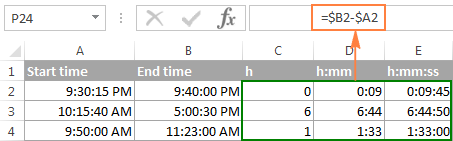Note. If the elapsed time is displayed as hash marks (#####), then either a cell with the formula is not wide enough to fit the time or the result of your time calculations is a negative value.

#### Formula 2. Calculating time difference with the TEXT function

Another simple technique to calculate the duration between two times in Excel is using the TEXT function:

• Calculate hours between two times:

`=TEXT(B2-A2, "h")`

• Return hours and minutes between 2 times:

`=TEXT(B2-A2, "h:mm")`

• Return hours, minutes and seconds between 2 times:

`=TEXT(B2-A2, "h:mm:ss")`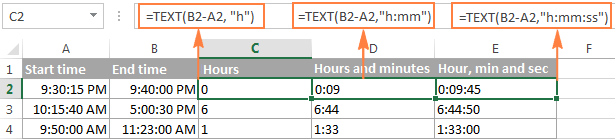Notes:

• The value returned by the TEXT function is always text. Please notice the left alignment of text values in columns C:E in the screenshot above. In certain scenarios, this might be a significant limitation because you won't be able to use the returned "text times" in other calculations.
• If the result is a negative number, the TEXT formula returns the #VALUE! error.

#### Formula 3. Count hours, minutes or seconds between two times

To get the time difference in a single time unit (hours ,minutes or seconds), you can perform the following calculations.

Calculate hours between two times:

To present the difference between two times as a decimal number, use this formula:

=(End time - Start time) * 24

Supposing that your start time is in A2 and end time in B2, you can use a simple equation B2-A2 to calculate the difference between two times, and then multiply it by 24, which is the number of hours in one day:

`=(B2-A2) * 24`

To get the number of complete hours, use the INT function to round the result down to the nearest integer:

`=INT((B2-A2) * 24)`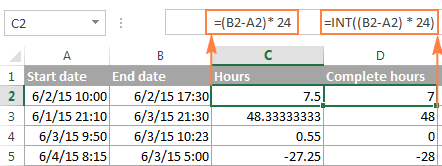Total minutes between two times:

To calculate the minutes between two times, multiply the time difference by 1440, which is the number of minutes in one day (24 hours * 60 minutes = 1440).

=(End time - Start time) * 1440

As demonstrated in the following screenshot, the formula can return both positive and negative values, the latter occur when the end time is less than the start time, like in row 5:

`=(B2-A2)*1440`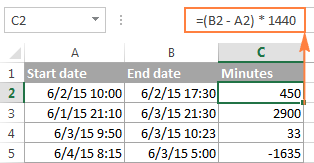Total seconds between times:

To get the total seconds between two times, you multiply the time difference by 86400, which is the number of seconds in one day (24 hours * 60 minutes * 60 seconds = 86400).

=(End time - Start time) * 86400

In our example, the formula is as follows:

`=(B2-A2)* 86400`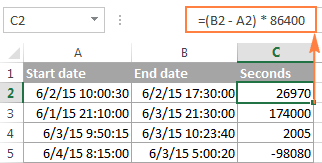Note. For the results to display correctly, the General format should be applied to the cells with your time difference formula.

#### Formula 4. Calculate difference in one time unit ignoring others

To find the difference between 2 times in a certain time unit, ignoring the others, use one of the following functions.

• Difference in hours, ignoring minutes and seconds:

`=HOUR(B2-A2)`

• Difference in minutes, ignoring hours and seconds:

`=MINUTE(B2-A2)`

• Difference in seconds, ignoring hours and minutes:

`=SECOND(B2-A2)`

When using Excel's HOUR, MINUTE and SECOND functions, please remember that the result cannot exceed 24 for hours and 60 for minutes and seconds.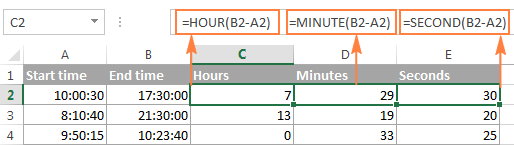Note. If the end time is less than the start time (i.e. the result of the formula is a negative number), the #NUM! error is returned.

#### Formula 5. Calculate elapsed time from a start time to now

In order to calculate how much time has elapsed since the start time to now, you simply use the NOW function to return today's date and the current time, and then subtract the start date and time from it.

Supposing that the beginning date and time is in call A2, the formula below returns the following results, provided you've applied an appropriate time format to column B, h:mm in this example:

`=NOW()-A2`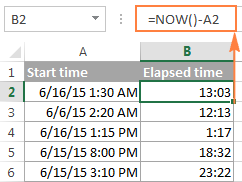In case the elapsed time exceeds 24 hours, use one of these time formats, for example d "days" h:mm:ss like in the following screenshot: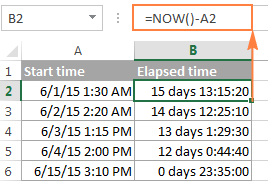If your starting points contain only time values without dates, you need to use the TIME function to calculate the elapsed time correctly. For example, the following formula returns the time elapsed since the time value in cell A2 up to now:

`=TIME(HOUR(NOW()), MINUTE(NOW()), SECOND(NOW())) - A2`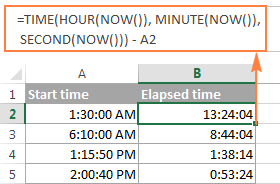Note. The elapsed time is not updated in real-time, it refreshes only when the workbook is reopened or recalculated. To force the formula to update, press either Shift + F9 to recalculate the active spreadsheet or hit F9 to recalculate all open workbooks.

#### Formula 5. Display time difference as "XX days, XX hours, XX minutes and XX seconds"

This is probably the most user-friendly formula to calculate time difference in Excel. You use the HOUR, MINUTE and SECOND functions to return corresponding time units and the INT function to compute the difference in days. And then, you concatenate all these functions in a single formula along with the text labels:

`=INT(B2-A2) & " days, " & HOUR(B2-A2) & " hours, " & MINUTE(B2-A2) & " minutes and " & SECOND(B2-A2) & " seconds"`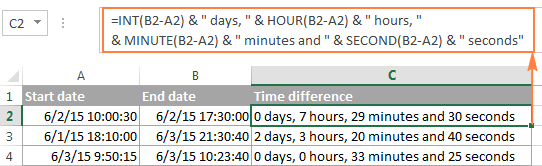To instruct your Excel time difference formula to hide zero values, embed four IF functions into it:

`=IF(INT(B2-A2)>0, INT(B2-A2) & " days, ","") & IF(HOUR(B2-A2)>0, HOUR(B2-A2) & " hours, ","") & IF(MINUTE(B2-A2)>0, MINUTE(B2-A2) & " minutes and ","") & IF(SECOND(B2-A2)>0, SECOND(B2-A2) & " seconds","")`

The syntax may seem excessively complicated, but it works :)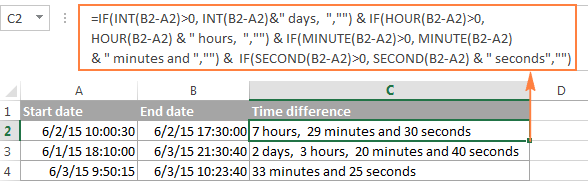Alternatively, you can calculate time difference by simply subtracting the start time from the end time (e.g. `=B2-A2`), and then apply the following time format to the cell:

d "days," h "hours," m "minutes and" s "seconds"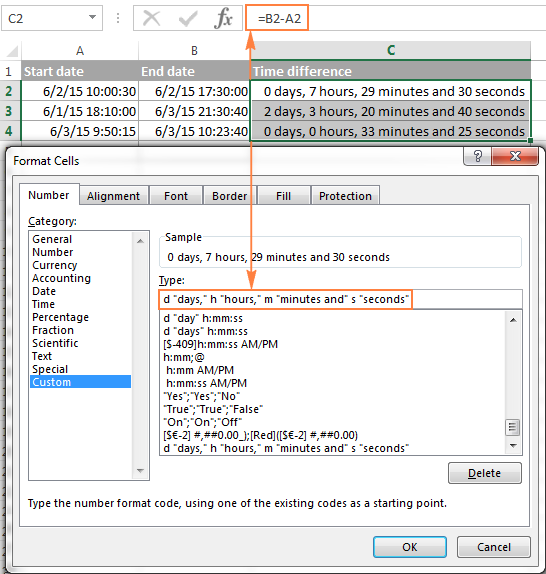An advantage of this approach is that your result would be a normal time value that you could use in other time calculations, while the result of the complex formula discussed above is a text value. A drawback is that the custom time format cannot distinguish between zero and non-zero values and ignore the latter. To display the result in other formats, please see How to show time over 24 hours, 60 minutes, 60 seconds.

## How to calculate and display negative times in Excel

When calculating the time difference in Excel, you may sometimes get the result as ###### error because the difference is a negative time. But is there a way to show negative times properly in Excel? Of course, there is a way, and even more than one :)

#### Method 1. Change Excel Date System to 1904 date system

The fastest and easiest way to display negative time normally (with a minus sign) is switching to the 1904 date system. To do this, click File > Options > Advanced, scroll down to the When calculating this workbook section and put a tick in the Use 1904 date system box.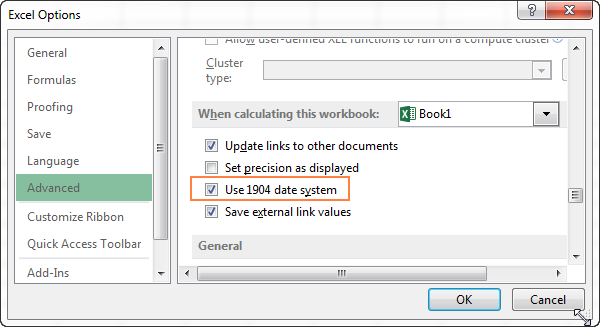Click OK to save the new settings, and from now on negative times will be displayed correctly, like negative numbers: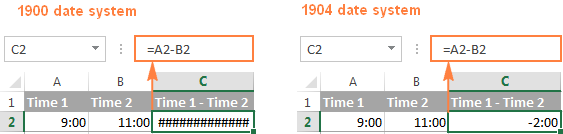#### Method 2. Calculate negative time in Excel with formulas

Is changing Excel's default Date System is not an option, then you can force negative times to display properly using one of the following formulas:

`=IF(A2-B2>0, A2-B2, "-" & TEXT(ABS(A2-B2),"h:mm"))`

`=IF(A2-B2>0, A2-B2, TEXT(ABS(A2-B2),"-h:mm"))`

Both formulas check if the time difference (A2-B2) is greater than 0, and if it is they return that difference. If the time difference is less than zero, the first formula calculates the absolute difference and concatenates the minus sign. The second formula yields exactly the same result by using a negative time format "-h::mm".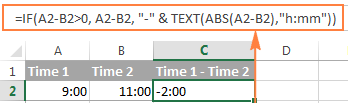Note. Please keep in mind that unlike the first method that treats negative times as negative numeric values, the result of the TEXT function is always a text string that cannot be used in calculations or other formulas.

## Adding and subtracting time in Excel

Basically, there are 2 ways to add and subtract time in Excel:

• Using the TIME function
• Using arithmetic calculations based on the number of hours (24), minutes (1440) and seconds (86400) in one day

The `TIME(hour, minute, second)` function makes Excel time calculations really easy, however it does not allow adding or subtracting more than 23 hours, or 59 minutes, or 59 seconds. If you are working with bigger time intervals, then use one of the arithmetic calculations demonstrated below.

### How to add or subtract hours to time in Excel

To add hours to a given time in Excel, you can use one the following formulas.

TIME function to add under 24 hours

=Start time + TIME(N hours, 0, 0)

For example, if your start time is in cell A2, and you want to add 2 hours to it, the formula is as follows:

`=A2 + TIME(2, 0, 0)`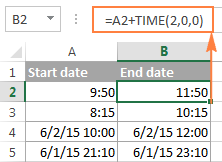Note. If you try adding more than 23 hours with the TIME function, the specified hours will be divided by 24 and the remainder will be added to the start time value. For example, if you try to add 25 hours to "6/2/2015 10:00 AM" (cell A4) using the formula `=A4 + TIME(25, 0, 0)`, the result will be "06/02/2015 11:00", i.e. A4 + 1 hour.

Formula to add any number of hours (under or over 24 hours)

The following formula has no limitations to the number of hours you want to add:

= Start time + (N hours / 24)

For example, to add 28 hours to the start time in cell A2, enter the following formula:

`=A2 + (28/24)`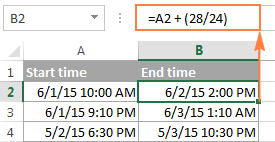To subtract hours from a given time, you use analogous formulas, and just replace "+" with the minus sign:

For example, to subtract 3 hours from the time in cell A2, either of the following formulas will do:

`=A2-(3/24)`

`=A2-TIME(3,0,0)`

To subtract more than 23 hours, use the first one.

### How to add / subtract minutes to time in Excel

To add minutes to a given time, employ the same techniques that we've just used for adding hours.

To add or subtract under 60 minutes

Use the TIME function and supply the minutes you want to add or subtract in the second argument:

=Start time + TIME(0, N minutes, 0)

And here are a couple of real-life formulas to calculate minutes in Excel:

To add 20 minutes to the time in A2: =A2 + TIME(0,20,0)

To subtract 30 minutes from the time in A2: =A2 - TIME(0,30,0)

To add or subtract over 60 minutes

In your calculation, divide the number of minutes by 1440, which is the number of minutes in a day, and add the quotient to the start time:

=Start time + (N minutes / 1440)

To subtract minutes from time, simply replace plus with the minus sign. For example:

To add 200 minutes: `=A2 + (200/1440)`

To subtract 300 minutes: `=A2 -(300/1440)`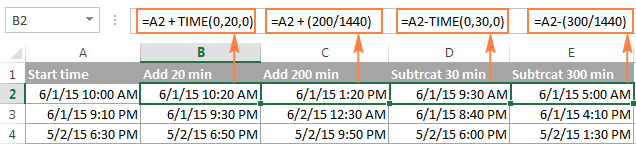### How to add / subtract seconds to a given time

Second calculations in Excel are done in a similar fashion.

To add under 60 seconds to a given time, you can use the TIME function:

=Start time + TIME(0, 0, N seconds)

To add more than 59 seconds, use the following formula:

=Start time + (N seconds / 86400)

To subtract seconds, utilize the same formulas with the minus sign (-) instead of plus (+).

In your Excel worksheets, the formulas may look similar to these:

To add 30 seconds to A2: `=A2 + TIME(0,0,31)`

To add 1200 seconds to A2: `=A2 + (1200/86400)`

To subtract 40 seconds from A2: `=A2 - TIME(0,0,40)`

To subtract 900 seconds from A2: `=A2 - (900/86400)`

## How to sum time in Excel

The Excel sum time formula is the usual SUM function, and applying the proper time format to the result is what does the trick.

Supposing you have a few project times in column B and you want to add them up. You write a simple SUM formula like the one below and get the result in the default format such as hh:mm:ss.

`=SUM(B2:B4)`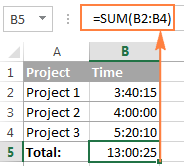In some cases the default time format works just fine, but sometimes you may want more, for example to display the total time as minutes and seconds, or seconds only. The good news is that no other calculations are required, all you have to do is apply custom time format to the cell with the SUM formula.

Right click the cell and select Format Cells in the context menu, or press Ctrl + 1 to open the Format Cells dialog box. Select Custom from the Category list and type one of the following time formats in the Type box:

• To display total time as minutes and seconds: [m]:ss
• To display total time as seconds: [ss]

The result will look as follows: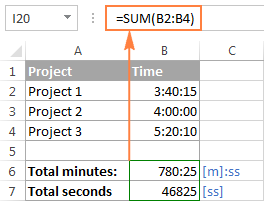### How to sum over 24 hours in Excel

In order to add up more than 24 hours, you use the same SUM formula as discussed above, and apply one of the following time formats to the cell:

 Format Displays as Explanation [h]:mm 30:10 Hours and minutes [h]:mm:ss 30:10:20 Hours, minutes and seconds [h] "hours", mm "minutes", ss "seconds" 30 hours, 10 minutes, 20 seconds d h:mm:ss 1 06:10:20 Days, hours, minutes and seconds d "day" h:mm:ss 1 day 06:10:20 d "day," h "hours," m "minutes and" s "seconds" 1 day, 6 hours, 10 minutes and 20 seconds

To see how these custom time formats may look like in your Excel worksheet, please have a look at the screenshot below, where the same SUM formula is entered in cells A9 to A13:

`=SUM(\$B\$2:\$B\$4)`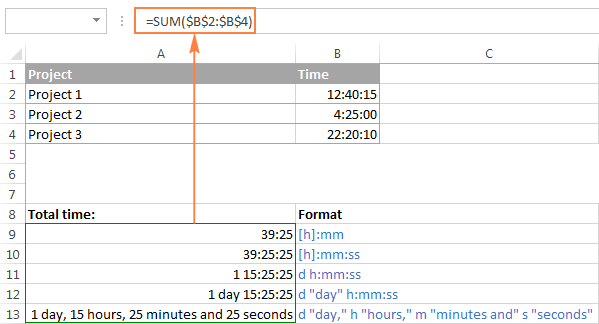Note. The above custom time formats work for positive values only. If the result of your time calculations is a negative number, e.g. when you are subtracting a bigger time from a smaller time, the result will be displayed as #####. To display negative times differently, please see custom format for negative time values.

Also, please keep in mind that the time format applied to a cell changes only the display presentation without changing the cell's value. For example, in the screenshot above, cell A13 looks like text, but in fact it's a usual time value, which is stored as a decimal in the internal Excel system. Meaning, you are free to refer to that cell in other formulas and calculations.

For more information, please see How to calculate and show over 24 hours, 60 minutes, 60 seconds.

## Date & Time Formula Wizard - quick way to calculate times in Excel

Now that you know a bunch of different formulas to add and subtract times in Excel, let me show you the tool that can do it all. Okay, almost all :)

Here comes Ablebit's Date & Time Formula Wizard for Excel:In the Date & Time Wizard dialog window, you switch to the Add or Subtract tab, depending on which operation you want to perform, and do the following:

• Click the Show time fields link in the left part of the window.
• Supply values or cell references for the formula arguments. As you fill in the argument boxes, the wizard builds the formula in the selected cell.
• When finished, click the Insert Formula

That's it! For example, this is how you can add the specified number of hours, minutes and seconds to the time in A4: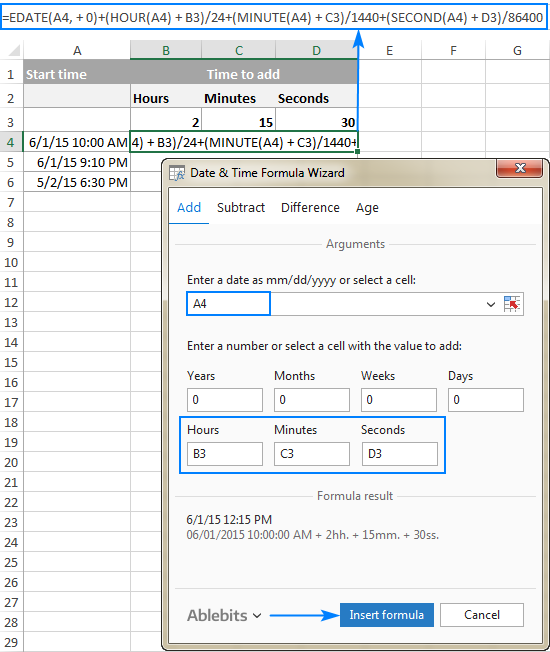If you need to copy the formula to other cells, fix all references except the cell containing the original time (A4) with the \$ sign like shown in the screenshot below (by default, the wizard always uses relative references). Then double-click the fill handle to copy the formula down the column and you are good to go!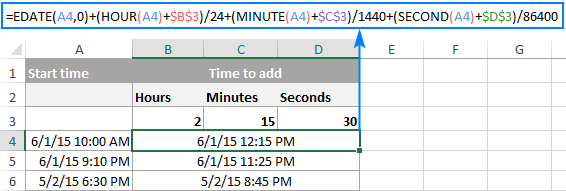Besides time calculations, the wizard can also add and subtract dates, get the difference between two dates, and calculate age from the birthdate.

If you are curious to try this tool in your own worksheets, you are welcome to download the evaluation version of our Ultimate Suite below.

This is how you calculate time in Excel worksheets. To learn other ways to manipulate dates and times in Excel, I encourage you to check out the resources at the end of this article. I thank you for reading and hope to see you on our blog next week!

Excel Time Calculations - formula examples (.xlsx file)
Ultimate Suite - trial version (.exe file)

### Excel Date and Time tutorials:

1. Setting up a time sheet. I have worked out the formula for daily hours worked and a weekly total, what I cant work out is one for the following.
total hours if greater than 37 or lesser than with a value in hours and minutes.
I can get the correct figure if the total hours are greater than 37 using =D7-TIME(37,0,)but when it goes into a negative the result is hours, mins subtracted from 24.
What do I need to do to the formula to just show negative hours,mins?????

2. Hi, I need to calculate the difference in time. I have my D&C columns formatted as "Time", and am trying the formula =MINUTE(D2-C2), but it just gives me #VALUE. Are there any other settings I need to check?

Here is what my data looks like in each column:
09/05/2019 3:41 PM 09/05/2019 3:57 PM

3. in excel i am only count between 3 cell time but when I am going to + more then 3 cell time then the value gose wrong ...

4. Hi may I ask anyone the formula to add restdays on work schedule? Thanks

5. I am trying to have a column which shows a "-" minus sign if two times produce a minus. ie. 00:00:50 & 00:00:48 = -00:02 or if the sum was 00:00:48 & 00:00:50 = (+)00:02 but using one sum not having to individually go through & tell the formula if the sum is a plus or minus result. The first timing is 50 secs & the second 48 secs then the result is -2 seconds, etc.

6. You have a great series of Excel lessons here.

I’m looking at the table right above the Formula 2 heading using the formula

=\$B2-\$A2

I noticed the calculations give incorrect totals.

Using line 3 in the table the correct number of hours should be 7 and not 6.

I can’t even figure out where the 44 minutes came from since 15 would be the quarter hour it should be 45 minutes. But really it should still be 15 minutes since we’re subtracting 15 minutes from nothing.

And lastly 30 seconds from 40 seconds leaves 10 seconds, where does 50 come from?

I’m trying to setup a time sheet if the form of:

Date In Out In Out Total

I know that my formula will be (Out1-In1)+(Out2-In2)=Total for the Day,
But I can’t seem to get past the incorrect calculations.

Is there some tid-bit of information that I’m missing or just not understanding?

Any help would be greatly appreciated.

7. Hi can someone help me with this formula, I am calculating time on excel with this formula but times are coming out as negative figures,
=IF(C33="","", (C33-C32)*24- C34)

8. Hello, I am trying to make a time sheet. Here is the example:
Start time: 12:30 am
Regular hours: 4.5 hours
Extra hours: 40 minutes
Break: 30 minutes if total hours more than 5 hours
Finish Time: ??? I need the formula which should be 6:10 am as total hour more than 5.
Total hours: 5.16 hours

9. Thank you so very much for an excellent article.
I haven't gotten my mind completely around it yet , but it certainly has me heading in the direction I need to go where as before I simply had no idea!

Thanks again ....5 Stars

10. I am trying to create a time sheet.
There will be 2 different in and out columns. I want a sum of all hours worked.
Example
A2 B2 C2 D2 E2
In Out In Out Total hours worked
7:00 A 8:30 A 11:00 A 5:00 P

11. I have an excel worksheet in which I'd like the date to update to the next date at a specific time. I have tried =today()+20/24, that only shows the current date and ten o clock in the evening. I am after it keep the previous data value (date) untill it passes a certain time value.
Lets say its 5:59 AM the date it shows in the cell is previous days date. At 6:00 AM it changes to the current days date. Like first at 5:59 its 14th of june and then at 6:00 its 15th of june.

12. Really very useful & excellent.

13. Really very useful & thank you so much.

14. Dear Friends
I want to write the calculated days.,Hours, Minutes in 00 digit format (Eg. 01 Days, 01 Hours, 01 Minutes) by using the below formula =IF(INT(B2-A2)>0, INT(B2-A2) & " days, ","") & IF(HOUR(B2-A2)>0, HOUR(B2-A2) & " hours, ","") & IF(MINUTE(B2-A2)>0, MINUTE(B2-A2) & " minutes and ","") & IF(SECOND(B2-A2)>0, SECOND(B2-A2) & " seconds","")

15. Hi,
I'm trying to calculate the amount of time left in a 24 hour period for multiple time slots that indicate dead time (time that is unavailable). The slots can have overlapping times. For ex. slot 1 = 8am-10am, slot 2 = 9am-11am, slot 3 = 7am-8:30am, slot 4 = 12pm-4pm, slot 5 = 1am-3am, slot 6 = 2:30 - 4:30pm. The slots do not have to be in chronological order. The result should be 24-10.5 = 13.5 left. Can anyone help?
Thanks

16. I want to know the formula to check whether for example 7:12pm lies between 11:45am, 12:00pm and 6:45pm, 7:30pm?

17. Here's a simple one but sleep deprivation may be causing me to overlook the solution...

Goal: TOTAL TIME WORKED LESS 30 MINUTE LUNCH (result cell: C1) using only cells for TIME IN, TIME OUT, AND HOURS WORKED.

Data Format: A1,B1,C1: TIME(Hour(01):Minute(01))
A1: IN TIME | B1: OUT TIME | C1: HOURS WORKED (LESS LUNCH)

Data Entered (Multiple variations):
1a. A1: 16:30 | B1: 23:00 | C1: =(B1-A1)-30 | RESULT: C1: 06:30 (0:30:00 not subtracted)
1b. A1: 16:30 | B1: 23:00 | C1: =(B1-A1)-00:30:00 | RESULT: C1: ERROR

Notes:
1. entering "-30" appeared to have no effect (changing to any number produced same result - always 06:30)
2. value in duration format '00:30:00' resulted in ERROR, adding parenthesis '(00:30:00)' had no effect, still ERROR

Eventually, and reluctantly, I added a column for LUNCH DURATION... which worked.
Data Format: A1,B1,D1: TIME(Hour(01):Minute(01)) | C1: DURATION(00:00:00)
A1: IN TIME | B1: OUT TIME | C1: LUNCH DURATION | D1: HOURS WORKED (LESS LUNCH)
A1: A1: 16:30 | B1: 23:00 | C1: 00:30:00 | D1: =(B1-A1)-C1 | RESULT: C1: 06:00

I didn't want to have a column for LUNCH DURATION. I feel it is unnecessary. I would rather just include the LUNCH DURATION in the formula.

How can I successfully achieve this without creating a column for LUNCH DURATION and using the column name in the formula?

18. Plz help me in excel sheet i want total no of hours worked formula

• See below..

19. I got x-numbers of objects with different timestamps during a 24h period.
I have the total time (End time - Start time) summed up, BUT i can't count the same time twice.
Example:
AAA 03/07/2019 09:40-10:15, 11:30-12:00 (95 minutes)
BBB 03/07/2019 09:40-10:20, 18:00-19:00 (100 minutes)
But there are 20 minutes during that day that have the same time and i can't just sum that up, so the total time should be 175 minutes.
1. How do i find the minutes that overlaps (i need to verify from the date).
2. How do i remove the duplicates.

20. If 06:00:00 to 14:00:00=A, 14:00:01 to 22:00:00=B Shift and other C-Shift how to calculate in between how many hours are in a Date in A Shift and how many hours in B Shift
Exam :
Start Date Start Time End Date End Time
31-05-2019 04:00:00 01-06-2019 09:00:00 = on 31.05.19 : C=2 and
01.06.19 : A=3

21. How can I calculate the time along with date plz help.

22. pls help me to calculate ETA, for example one has its ETA of 10 hours, and it comes back within 12 hours, second if vehicle is moving at 2300hrs and comes back next day at 0300 hrs, how will I calculate its ETA?

23. Hi All,
Good Day!
Need some help, i want to subtract two time and result in ms? Is there any possibility?
Start: 24/06/2019 6:37:06:06
End: 24/06/2019 6:37:06:06
currently i using this formula:
=IF(INT(H74-G74)>0, INT(H74-G74) & " days, ","") & IF(HOUR(H74-G74)>0, HOUR(H74-G74) & " hours, ","") & IF(MINUTE(H74-G74)>0, MINUTE(H74-G74) & " minutes and ","") & IF(SECOND(H74-G74)>0, SECOND(H74-G74) & " seconds","")

24. Date 1/5/2019 02/5/2019
Durations 01:10 03:58
any simple formula for above if exceed 3 hrs than burst SLA

25. Desperately need formula to calculate hours and minutes worked in a day. Also need formula for total hours and minutes worked in a week for payroll.

26. I have 2 times column
start time
end time
Now I want to calculate
How much hour is there between (6 A.M to 18 P.M) from those 2 starts and end column
How much hour is there between (18 P.M to 00:00 A.M) from those 2 starts and end column
How much hour is there between (00:00 A.M to 6:00 A.M) from those 2 starts and end column

27. a clock was correctly set at 12pm.when the exact time was 10:00 p.m. the clock shows 1910 p.m. find the correctly time when the clock shows at 11:00 p.m.?

28. How to Find in between time (12:32:00 PM to 01:12:00 AM)

29. Need a formula that compares a date and time stamp between two columns. If 1 column is below the date and time in the 2nd column then it would say "Yes","No" based on the snapshot. Any ideas? Date and time stamp are shown in the same column. Does this need to be broken down?

30. Hi,
I tried to used all possible formula to calculate time difference , but the return is Error
I have used formula =\$I2-\$H2 or =INT(I4-H4) & " days, " & HOUR(I4-H4) & " hours, " & MINUTE(I4-H4) & " minutes and " & SECOND(I4-H4) & " seconds"
Both gave me Error
Here is example:
Start Time End Time MTBF
4/29/19 18:24:10 4/29/19 23:19:56 #VALUE! (use formula =\$I2-\$H2 or

• I think I find the answer...
I use simple combine formula =A2+B2 , instead of formula =TEXT(A2,"m/dd/yy ")&TEXT(B2,"hh:mm:ss") , then the time difference formula =INT(D2-C2) & " days, " & HOUR(D2-C2) & " hours, " & MINUTE(D2-C2) & " minutes and " & SECOND(D2-C2) & " seconds" is working perfectly.

32. hi,
how to calculate the target time is 7 hours and start time is 7 am end time is 12:30 pm.
in this case how to calculate the how is complete the below 7 hrs and how is complete above 7hrs

33. Downtime in % per day = Down time of affected machine / Total Available time in a Day
=G5*H5*0.5/(47*24*60)

now please let me understand what is this 47
24 is hr,60 is min but what is 47 ?

34. Hi,
How can i calculate differnce in a tasks (Start date and end date) considering only Business hrs in that task (10 Am to 7 Pm)

I need to calculate it under only BH

35. Sum of hours is not correctly shown: 09:20 + 08:45 + 09:22 + 08:56 + 06:08 should come to 42:31 but using the SUM function it calculates as 18:31. Why & How?

• Hi Siddharth,
To display times over 24 hours, a special number format [h]:mm should be applied to the formula cell. You can find the detailed explanation with step-by-step instructions and screenshots in this tutorial:
How to show over 24 hours, 60 minutes, 60 seconds in Excel

• Thank you Svetlana, it worked.

36. Hi,
Thank you, it was interesting and easy to learn. I shall come up some others doubts in Future.
Regards,
Sujay.BS

37. Hi all, I'm trying to make a document calculating amount of time spent over a work-day, in different time periods. An example could be an over-all time period from 18.30 to 05.30. Cell A should define time spent between 18.00 and 21.00, Cell B define time spent between 21.00 and 00.00 and Cell C define time spent between 00.00 and 06.00. The result will be Cell A = 2,5 hours, Cell B = 3 hours and Cell C = 5,5 hours. But what formula should I use to get to the results? In advance thanks a lot! Best, Sune.

38. Hi Team,
Can anyone resolve my problem, it would be great. Actually my shift timing is "6 pm to 3:30 am" IST. I am facing problem in weekend days (Saturday morning after 12:00 am when i m getting any request, then it is not calculating because saturday is a weekend but my shift timing is 6 pm to 3:30 am till saturday morning.
Curently i am using one formula for Day shift which is working 100% accurate if shift timing is 12 pm to 9:30 pm and G2 = Start date, J2 = End date.

=(((NETWORKDAYS(G2,J2)-1)*("21:30:00"-"12:00:00")+IF(NETWORKDAYS(J2,J2),MEDIAN(MOD(J2,1),"21:30:00","12:00:00"),"21:30:00")-MEDIAN(NETWORKDAYS(G2,G2)*MOD(G2,1),"21:30:00","12:00:00")))

39. I have a cell calculating time remaining until a task is completed. Is there a way for when hours remaining = 0 to hide the hour digit and only show minutes remaining?

40. Trying to create a formula to calculate total time in hours and minutes.

Then the total number of hours and minutes converted into 15 min increments.

So for example, total mins & hours 8.40 (8 hours 40 mins)
In units 8.75

Help!!!

41. Kindly provide me a formula for from time - 9:45:00 PM & To time 1:15:00 AM, want time difference?? Using =xX9-XX9 formula. getting results ########

• Charles,
Hash symbols are displayed if either the formula cell is not wide enough to accommodate the result or the result is a negative value. If the former, just make the cell wider. If the latter, please see How to calculate negative times in Excel

42. Hi,
Please can someone tell how to calculate total hours.
Starting Time - 23:05:00
End Time - 00:10:00

Thank you.

43. The examples and illustrations are really very helpful. Thanks for your kind help.

44. Dear Ms. Svetlana Cheusheva,
So many thanks for your nice article.
Thanks & Regards
Sunil Kumar Pandey

45. Hi,
I need to subtract minutes or hours from a given time with date change.

Example: given time: 5:00 (am), needed time: 6 hours earlier.
Problem: Excel returns -1 instead of 23 (11 pm). How can I go around it?

Thanks a lot!
Yan

46. Hello. I have a spreadsheet with 40,000 rows of 2 columns each. StartTime and DurationInSeconds. I am trying to figure out a way to show how many of them happened at the same time. The StartTime is formatted as "mm/dd/yyyy hh:mm:ss" and the duration is just the number of seconds. I've already added a 3rd column =A1+TIME(0,0,B1) to show the StopTime so I have StartTime and StopTime. Now I need to see how many times they coincide.

47. I need to average times for several days using military time. I have day 1=00:45, day 2=00:20, Day 3= 23:59. When I run the formula +Average(A1:A3) Excel gives me 08:21 as my answer. If I change 23:50 to 00:01 it gives me 00:22 Which is more realistic. I am averaging over a 24 hour day prior to mid-night is causing my problems. Any Ideas????

48. Hi,
In one cell, say (A1) I have time, and so in another cell, i applied the Formula (=A1+1). Why do i get the same time?
What does that numeric 1 imply?

49. Hi, I need to calculate the time between certain hours. So for example, the below shows start and end times, and I need to calculate the hours between 10am and 2pm only.
Start time 3/12/2018 9:30:00 am
End Time 3/12/2018 11:30:00am